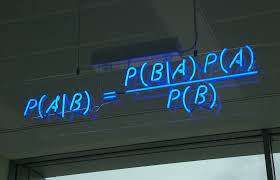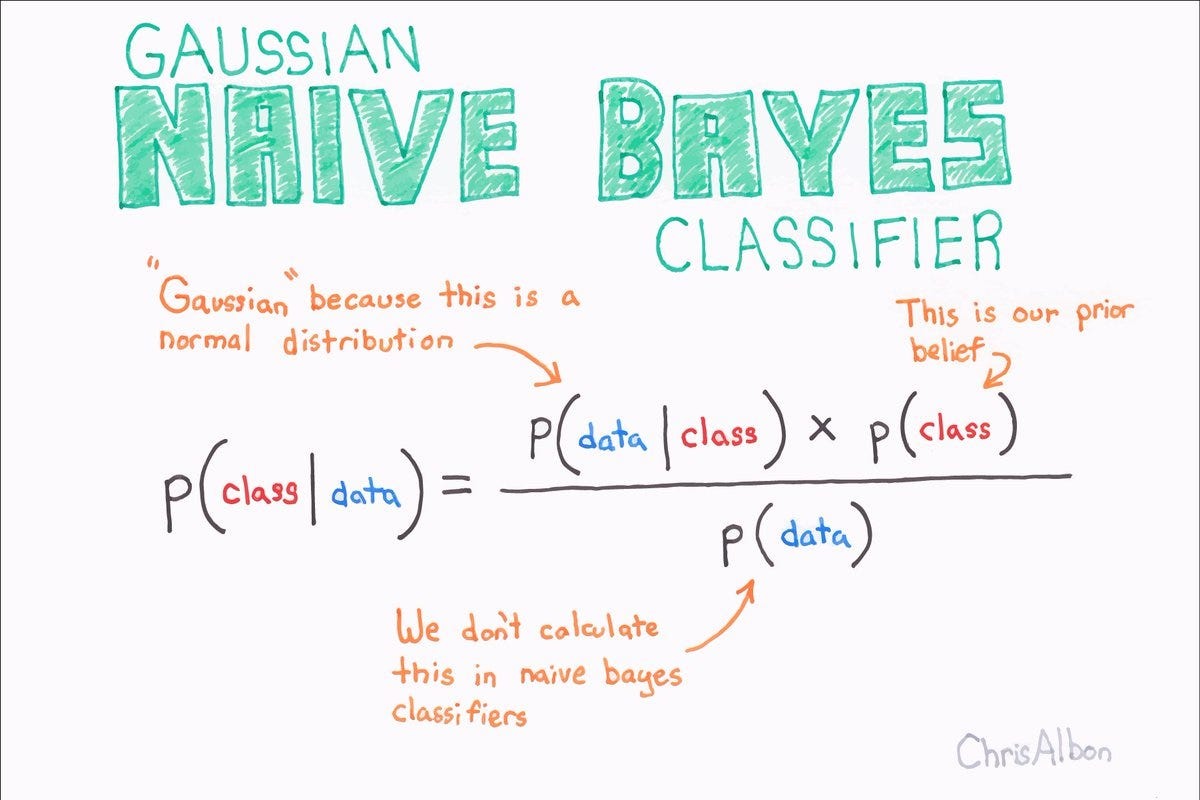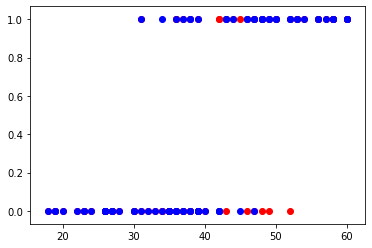0%
• Naive Bayes Intution
• What is Bayes Formulae
• Implementation Naive Bayes with socail network database

## What is Naive Bayes

In machine learning, naïve Bayes classifiers are a family of simple "probabilistic classifiers" based on applying Bayes' theorem with strong (naïve) independence assumptions between the features. They are among the simplest Bayesian network models. But they could be coupled with Kernel density estimation and achieve higher accuracy levels.

The Naive Bayesian classifier is based on Bayes’ theorem with the independence assumptions between predictors. A Naive Bayesian model is easy to build, with no complicated iterative parameter estimation which makes it particularly useful for very large datasets. Despite its simplicity, the Naive Bayesian classifier often does surprisingly well and is widely used because it often outperforms more sophisticated classification methods.

## Algorithm

Bayes theorem provides a way of calculating the posterior probability, P(c|x), from P(c), P(x), and P(x|c). Naive Bayes classifier assume that the effect of the value of a predictor (x) on a given class (c) is independent of the values of other predictors. This assumption is called class conditional independence.

## Bayes Formulae#### This can also be interpreted as## Implementation of Naive Bayes Classifier

### Import libraries

In :
import pandas as pd
import numpy as np
from matplotlib import pyplot as plt

In :
df = pd.read_csv('Social_Network_Ads.csv')
df.describe()

Out:
User ID Age EstimatedSalary Purchased
count 4.000000e+02 400.000000 400.000000 400.000000
mean 1.569154e+07 37.655000 69742.500000 0.357500
std 7.165832e+04 10.482877 34096.960282 0.479864
min 1.556669e+07 18.000000 15000.000000 0.000000
25% 1.562676e+07 29.750000 43000.000000 0.000000
50% 1.569434e+07 37.000000 70000.000000 0.000000
75% 1.575036e+07 46.000000 88000.000000 1.000000
max 1.581524e+07 60.000000 150000.000000 1.000000
In :
df.shape

Out:
(400, 5)
In :
df.head(5)

Out:
User ID Gender Age EstimatedSalary Purchased
0 15624510 Male 19 19000 0
1 15810944 Male 35 20000 0
2 15668575 Female 26 43000 0
3 15603246 Female 27 57000 0
4 15804002 Male 19 76000 0
In :
# here purchased is our dependent variable

In :
#Converting string values to int so that our model can fit to the dataset better
from sklearn.preprocessing import LabelEncoder
scall = LabelEncoder()
df.iloc[: , 1] = scall.fit_transform(df.iloc[:,1])

Out:
User ID Gender Age EstimatedSalary Purchased
0 15624510 1 19 19000 0
1 15810944 1 35 20000 0
2 15668575 0 26 43000 0
3 15603246 0 27 57000 0
4 15804002 1 19 76000 0
In :
# Splitting x and y here
x = df.iloc[: , 1:4].values
y = df.iloc[:, 4].values

print(x[:5])

[[    1    19 19000]
[    1    35 20000]
[    0    26 43000]
[    0    27 57000]
[    1    19 76000]]

In :
# train test split
from sklearn.model_selection import train_test_split
x_train , x_test , y_train , y_test = train_test_split(x, y , train_size = 0.8, test_size = 0.2 , random_state = 1)
print(x_train.shape, x_test.shape , y_train.shape)

(320, 3) (80, 3) (320,)


## Model fitting and predicting score

In :
from sklearn.naive_bayes import GaussianNB
classifier = GaussianNB()
classifier.fit(x_train, y_train)

Out:
GaussianNB(priors=None, var_smoothing=1e-09)
In :
#predicting values
y_pred = classifier.predict(x_test)


## Evaluating model prediction

In :
from sklearn.metrics import accuracy_score, confusion_matrix
print('accuracy oy model is : ', accuracy_score(y_test, y_pred))
print('Confusion Matrix:','\n', confusion_matrix(y_test, y_pred))

accuracy oy model is :  0.8625
Confusion Matrix:
[[41  7]
[ 4 28]]

In :
## we have 86% accuracy in our model

In :
plt.scatter(x_test[:, 1],y_test,color ='red')
plt.scatter(x_test[:,1],y_pred, color = 'blue')
plt.show()We can clearly see the results the graph The values that are in a different color are predicted wrong rest are right

In [ ]: